Non Verbal Reasoning - Mirror Images

Exercise :: Mirror Images - Section 1

In each of the following questions you are given a combination of alphabets and/or numbers followed by four alternatives (1), (2), (3) and (4). Choose the alternative which is closely resembles the mirror image of the given combination.

21.

Choose the alternative which is closely resembles the mirror image of the given combination.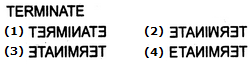A. 1 B. 2 C. 3 D. 4

Explanation:

No answer description available for this question. Let us discuss.

22.

Choose the alternative which is closely resembles the mirror image of the given combination.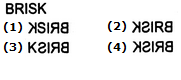A. 1 B. 2 C. 3 D. 4

Explanation:

No answer description available for this question. Let us discuss.

23.

Choose the alternative which is closely resembles the mirror image of the given combination.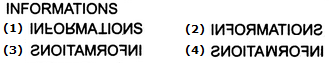A. 1 B. 2 C. 3 D. 4

Explanation:

No answer description available for this question. Let us discuss.

24.

Choose the alternative which is closely resembles the mirror image of the given combination.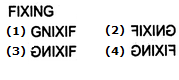A. 1 B. 2 C. 3 D. 4

Explanation:

No answer description available for this question. Let us discuss.

25.

Choose the alternative which is closely resembles the mirror image of the given combination.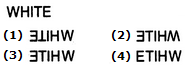A. 1 B. 2 C. 3 D. 4